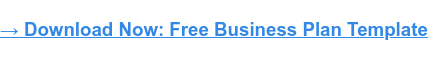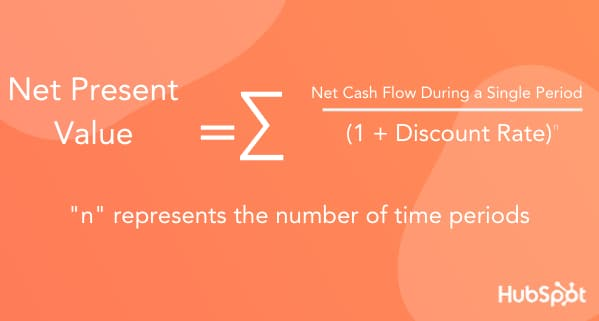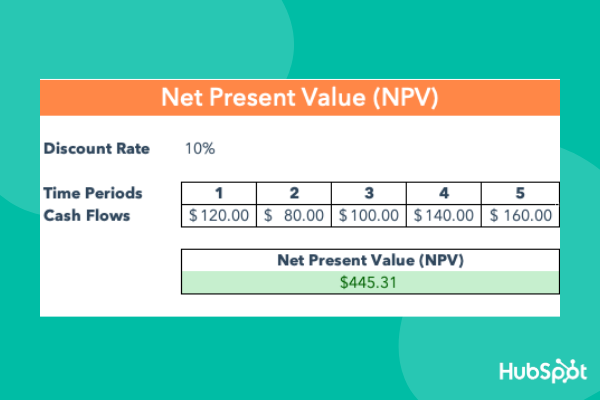Did you know Warren Buffet bought his first stock at 11 years old? Oh, and he filed for taxes at 13. I don't know about you, but at 11, my biggest goal was getting my Mom to serve pizza for dinner.

Buffett is an investor, business magnate, and philanthropist who's known as one of the most successful investors of all time He's used decades of experience to grow his wealth and further sharpen his investing prowess. And he concisely sums up the process of evaluating investments with this quote:

"Leaving aside tax factors, the formula we use for evaluating stocks and businesses is identical. Indeed, the formula for valuing all assets that are purchased for financial gain has been unchanged since it was first laid out by a very smart man in about 600 B.C. (though he wasn’t smart enough to know it was 600 B.C.)."

So, what is this ancient formula? And how does Warren Buffett evaluate investment opportunities?

He uses net present value (NPV).

While I can't promise you'll be the next Warren Buffet, I can tell you more about net present value and how it's used to identify great investments. Let's dive in.The net present value method is used to evaluate current or potential investments and allows you to calculated the expected return on investment (ROI) you'll receive.This video from the Harvard Business Review provides a brief overview of net present value.

NPV is calculated using the following formula:Here's the written formula:

Net Present Value (NPV) = Cash flow / (1 + discount rate) ^ number of time periods

When there are multiple periods of projected cash flows, this formula is used to calculate the PV for each time period. Then investors or analysts sum the values, and the initial investment is subtracted from the sum to get the net present value (NPV).

The discount rate that's used depends on the company and how it gets its funding. For example, if shareholders expect a 10% return on investment, the business will often use that percentage as the discount rate.

If the net present value is positive, your project is profitable. But, if the NPV is negative, your project is unprofitable. When comparing multiple projects, the one with the largest NPV will provide the highest return.

In summary, net present value translates the amount of money you expect to make from an investment into today's dollars.

## Net Present Value Example

Ready to see net present value in action? Let's say Pet Supply Company is comparing two projects to invest in. The discount rate for both projects is 10%.

### Project 1

• Initial investment: \$10,000
• Discount rate: 10%
• Year 1: \$5,000
• Year 2: \$15,000
• Year 3: \$9,000
• Year 4: \$18,000

Let’s calculate the present values for each year of the project:

• Year 1: 5,000/(1 + .10)^1 = \$4,545
• Year 2: 15,000/(1 + .10)^2 = \$12,397
• Year 3: 9,000/(1 + .10)^3 = \$6,762
• Year 4: 18,000/(1 + .10)^4 = \$12,294

Find the summation of these present values and subtract the initial investment amount to find the net present value.

NPV = (\$4,545 + \$12,397 + \$6,762 + \$12,294) - \$10,000

NPV = \$25,998

### Project 2

• Initial investment: \$5,000
• Discount rate: 10%
• Year 1: \$8,000
• Year 2: \$16,000

Let’s calculate the present values for each year of the project:

• Year 1: 8,000/(1 + .10)^1 = \$7,273
• Year 2: 16,000/(1 + .10)^2 = \$13,223

Here is the resulting formula after the present value is calculated for each cash flow and time period:

NPV = (\$7,273 + \$13,223) - \$5,000

NPV = \$15,496

The NPV for Project 1 is \$25,998 and Project 2 is \$15,496. Since Project 1 has a higher NPV value, Pet Supply Company should invest in that project.

So, how can you perform an NPV analysis? Luckily, you can crunch the numbers in Excel. Here are the steps to do so.

1. Determine the discount rate and add it to a cell.
2. Add the number of time periods in consecutive order.
3. Enter the expected cash flows for each time period.
4. Calculate NPV by typing the following Excel formula in a new cell: =NPV(select the discount rate cell, select first cash flow cell:last cash flow cell)Source: HubSpot

When using the NPV formula in Excel, you'll need to ensure that you've properly calculated the inputs so the resulting number is accurate. This is a quick method of calculating NPV, but more advanced financial modeling is often used to see each calculation and input that goes into the formula. Investors and analysts can see all the formulas used for the calculations which makes it easier to audit.

With net present value (NPV) you'll be able to effectively evaluate projects and investments to determine the return you'll receive from the investment. To learn more, check out this comprehensive guide to income statements next.Originally published Feb 9, 2020 7:45:00 PM, updated February 10 2020

Topics:

Sales Metrics